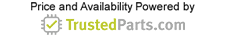Motor Control and Switchgear

# DC Motor Calculations—Part 1

21 April 2017

Direct-current motors transform energy created by electrical current to mechanical energy. It is an important component for industry today. For designers and engineers, it is also important to understand the working principle of DC motors, how to make calculations and how to select a DC motor. We can consider a DC motor in terms of the input-output relationship. The input parameters are the supply voltage (E) and the supply current (I) and the output mechanical parameters are the torque (T) and the rotational speed (ω). A representation of the functional block diagram is shown in Figure 1.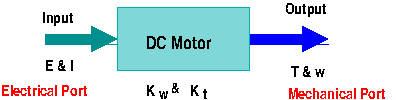Figure 1: DC motor block diagram.

The motor parameters, Kω and Kt, are called motor velocity constant and motor torque constant, respectively. These are specific parameters of a particular motor. Normally these parameters can be found in the motor specification data sheets of DC motors.

[Explore DC motor suppliers at GlobalSpec's Products directory.]

The energy conversion is accomplished by the well-known physics principle called motoring action: when a current carrying conductor is placed in a magnetic field, the conductor experiences a mechanical force. The direction of the force is determined by the Fleming's left hand rule as illustrated in Figure 2.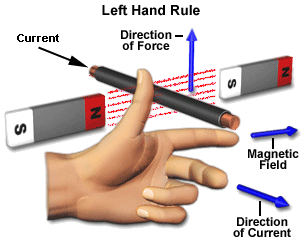Figure 2: Fleming’s left-hand rule. Source: www.electrical4u.com

The direction of the force generated by the magnetic field is perpendicular to both the direction of the current and the field. If we keep the index finger, the thumb and the middle finger perpendicular to each other, and if the middle finger represents the direction of the current and the index finger shows the direction of the magnetic field, then the thumb indicates the direction of the force. This is precisely what happens with the operation of a DC motor.

The main parts of a DC motor consist of a closed-loop conductor carrying the current called the armature, a permanent or an electromagnet (for typical DC motors), a battery or DC voltage source connected to the armature that produces the current through the armature, and brushes and a commutator to ease the rotation of the armature. Because the armature is located inside the magnetic field, once current flows through it, the generated force will actuate to force the armature to rotate. Figure 3 shows the diagram of the main parts of a typical brushed DC motor.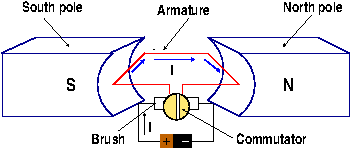Figure 3: Main parts of a DC motor.

Important definitions

Three basic parameters are important to understand motors in general: torque, angular speed and power. A short description of each follows.

Torque

Torque (also known as moment), etymologically derived from the Latin word twist, is defined as a force that produces or tends to produce rotation or torsion. A torque is created when a perpendicular force is applied at the end of a shaft, as is shown in the torque diagram below. The value of the torque is then given by the product of this force times the radius (the distance from the pivot to the location where the force is applied), also called the moment arm.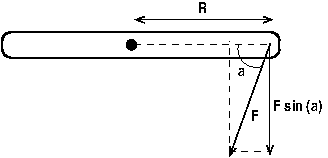Figure 4: The perpendicular component of F produces the torque.

A force F is applied to the shaft at an angle a, as shown. The perpendicular component of this force is Fsin(a) and the moment arm is R. Thus the torque is given by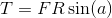The SI units of torque are newton-meter (N-m) and the English units are inch-pound (in-lb), feet-pound (ft-lb) or inch-ounce (in-oz). The following table shows some conversion units.

 SI English 1 N-m = 0.738 ft-lb 1 in-lb = 0.113 N-m 1 N-m = 0.113 in-lb 1 ft-lb = 1.356 N-m 1 N-m = 141.61 in-oz 1 in-oz = 7.062E-03 N-m

Rotational speed

Rotational or angular speed is measured in terms of the number of revolutions a shaft makes per unit time. The Greek letter omega, ω, is typically used to represent this quantity, and the units are radian/second (rad/s, SI unit), revolution/second (rps), or, among others, revolution/minutes (rpm). When using this parameter in calculations, we must use rad/s if all the other units are in the SI system, and degrees/sec if we are using the English system. An important conversion to remember is the relationship between rpm and rad/sec, given by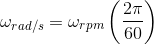It is also important to remember the following conversions:

 1 revolution = 360° 1 revolution = 2π radians 1 radian = 180/ π degrees 1 degree = π/180 radians

Power

In rotational motion, power is defined in terms of the torque, as follows

P = T ω

The standard SI unit of power is watts (W), which is equal to N-m/s, and in the English system we normally use ft-lb/s, or horsepower (hp). The following table shows some conversions:

 SI English 1 W = 1 N-m/s 1 ft-lb/s = 1.618 E-03 hp 1W = 0.738 ft-lb/s 1 ft-lb/s = 1.356 W 1 W = 1.341E-03 hp

Equivalent circuit of DC motors

A DC motor can be represented by the following circuit (Figure 5):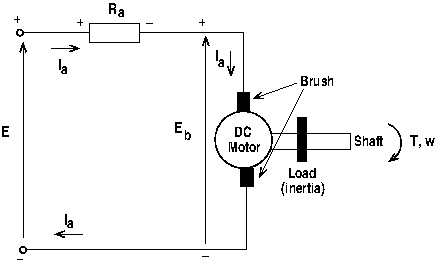Figure 5: DC motor representation.

The motor itself is represented by the circle surrounded by the brushes. The external power or power supply, E, is connected to the brushes through the armature. The armature is represented by its equivalent resistance, Ra. The current through the armature is Ia. The mechanical terminal (output) contains the shaft connected to the motor and the armature. In turns, the combination of armature and shaft is coupled to the mechanical load. When current is flowing through the armature, the magnetic field produces a torque, T, forcing the armature to rotate.

Back e.m.f.

As soon as the armature begins to rotate, a second phenomenon takes place. The armature rotating inside a magnetic field behaves like a generator, inducing an electromotive force (emf) voltage (Eb). This induced voltage, according to Lenz's law, acts in opposite direction to the applied (supply) voltage (E). The induced voltage is known as back or counter emf.

This induced voltage makes the DC motor a self-regulating machine, i.e., it makes the motor to draw as much armature current as is just sufficient to develop the torque required by the load. Back emf in a DC motor regulates the flow of armature current, i.e., it automatically changes the armature current to meet the load requirement.

The back emf value is proportional to the speed of rotation, like in a true generator, and to the flux per pole, and it is given by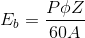where P is the number of poles, ϕ is the flux per pole, Z is the number of conductor, A is the number of parallel paths, and ω is the motor speed. With the exception of the motor speed, all other parameters in the equation are constants for a particular motor, thus we can write this equation as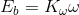The constant K ω, as we saw earlier, is called the motor speed constant.

Acceleration of the motor

An equation similar to the last equation can be developed relating the torque and the armature current. It happens that the torque is directly proportional to this current, and given by

T = KtIa

Where Kt is the motor torque constant.

The current flow through the armature is limited only by its resistance, and given by the Kirchhoff current law (KCL):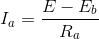At the very start of the motor ω = 0, and the induced voltage is Eb = 0. Then the starting current is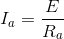Starter

The starting current is many times larger than the normal full-load rated current. Because the armature resistance of DC motors is very low, this starting current is dangerously large and can damage the motor windings and other parts of the motor. In order to avoid this, a DC motor must have a starter, which is nothing more than a variable resistance in series with the armature circuit. As the speed of the armature increases, the starter resistance is gradually reduced, so that the motor can attain full speed without the added starter resistance. To illustrate the importance of the starter, let's look at an example. Suppose you have a DC motor rated at 10 hp, 220 V and full load rated current of 25 A. The armature resistance is 0.5 Ω. Without a starter, when the power is applied to the motor, the initial current will be 220/0.5=440 A. This is 17.5 times the full-load current! So the need of a starter is imperative.

Speed regulation

An important feature of DC motors is their ability to maintain the same speed under variable load. To see how this is possible, let's assume that the motor is running at a speed of ω with a back e.m.f. of Eb. If the load is, for instance, increased, the speed will be reduced and Eb will decrease. Therefore the voltage difference E - Eb increases, and so does the armature current. With an increase in armature current, the torque will increase forcing an increase of the speed. This is similar to a closed-loop feedback system.

To complete this part of DC Motor Calculations, let's look at a simple problem.

Example

A DC motor is connected to a source of 150 V, and its armature resistance is 0.75 Ohms. The armature generates 40 V when running at a speed of 400 rpm. Calculate:

1) The starting current

2) The back e.m.f. when the motor runs at 800 rpm and when running at 1200 rpm

3) The armature current when running at the speeds in item 2

Solution

1) The starting current is given by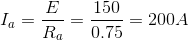2) We know that the back e.m.f. is directly proportional to the speed, then

Eb = 80 V at ω = 800 rpm

Eb = 120 V at ω = 1200 rpm

3) At ω = 880 rpm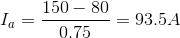and at ω = 1200 rpm the current is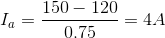Please keep checking Electronics360 for DC Motor Calculations—Part 2.